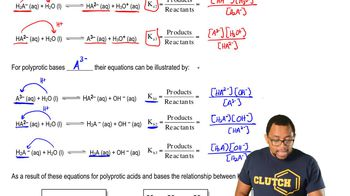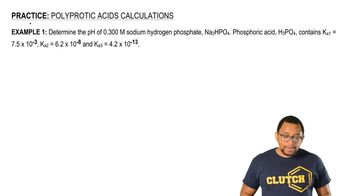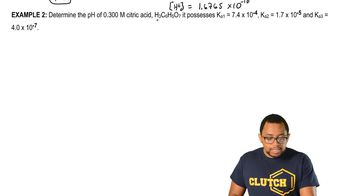Start typing, then use the up and down arrows to select an option from the list.# Polyprotic Acids

Jules Bruno
223views
1
determine the pH of 300 Mueller sodium hydrogen phosphate, which is N A to H P 04 Here, it says phosphoric acid, which is H three people, four, contains three K values given. All right, so we have to figure out the pH of this particular form. And to be able to solve this question, we have to look and see which one of the different forms could this possibly represent? So phosphoric acid represents the acidic form of our compound. Once it's given away its first H plus, it becomes H to peel four minus. So this is intermediate one. Once it's given away its second H plus, it becomes HP all four to minus. So this would be intermediate, too. And then, when it's given away its final H plus, it becomes peel for three minus, which is the basic form we have to look and see. Sodium Hydrogen phosphate represents which one of these four forms. So if we look, it's made up of what two and a plus, which is just spectator ions, which really don't matter, plus H p +04 to minus. So we can clearly see that this ion here represents this ion here, which means we're dealing with the second intermediate form. And remember, when it comes to calculating the pH of any one of the intermediate forms, we have to remember the equations associated with them. So for the second, intermediate H plus equals square, root off K two times K three times the initial concentration of the compound, plus que two times K W divided by K two plus the initial concentration of the compound. All right, so okay, a two is this number here, So plug it in que three. Is this value here? So plug it in. The initial concentration of the compound is 0. plus que two, which is still that number 6.2 times 10 to the negative, eight times K W, which is 1.0 times 10 to the negative divided by que two, which is 6.2 times 10 to the negative eight, plus the initial concentration again. All right, so multiply these three together, make sure you put them in parentheses. Multiply these two together, make sure you put them in parentheses, and then we're gonna add these together. So when we do piece by piece we're gonna get 7812 times 10 to the negative. 21 plus 6.2 times to the negative. 22 divided by when I add those two numbers together on the bottom, it gives me point 62 makes you put them in parentheses. When you add the two numbers on top, then divide by the number on the bottom. So if you do that correctly, you'll get 2. times 10 to the negative 20 and the square root of that, which will give us our H plus equals 1.6765 times 10 to the negative 10. So pH equals negative log of H plus, which we just found. So plug that in. So that's gonna give me 9.78 as the pH of my solution. So remember, it's tricky when it comes to Polly Protic. Acid or base is so you have to look and see which one of the four forms are we dealing with. If you're dealing with the acidic form, that means you're gonna do K one and do a nice chart to find H 30 plus concentration and from there, uh, ph If you're dealing with the intermediate one or two, they each have their own separate equation, which we talked about previously. And then if you're dealing with the basic form, that means we're dealing with cabe one. So we do a nice chart again. It would react with water. Water would donate an H plus to create O. H minus as a product. When we saw for X, we'd find the concentration of H minus. Taking the negative log of that would give us P O. H, which we then subtract from Ph to get our final answer. Most attract from 14 to find pH as our final answer. So, guys, these other approaches we need to take when it comes to these types of compounds. Not that we've seen this version. Take a look at the second one and see if maybe you can figure it out before we handle it together. And if you're stuck, don't worry. Just click over to the next video and see how I approach the next example03:0104:1204:0805:1806:56